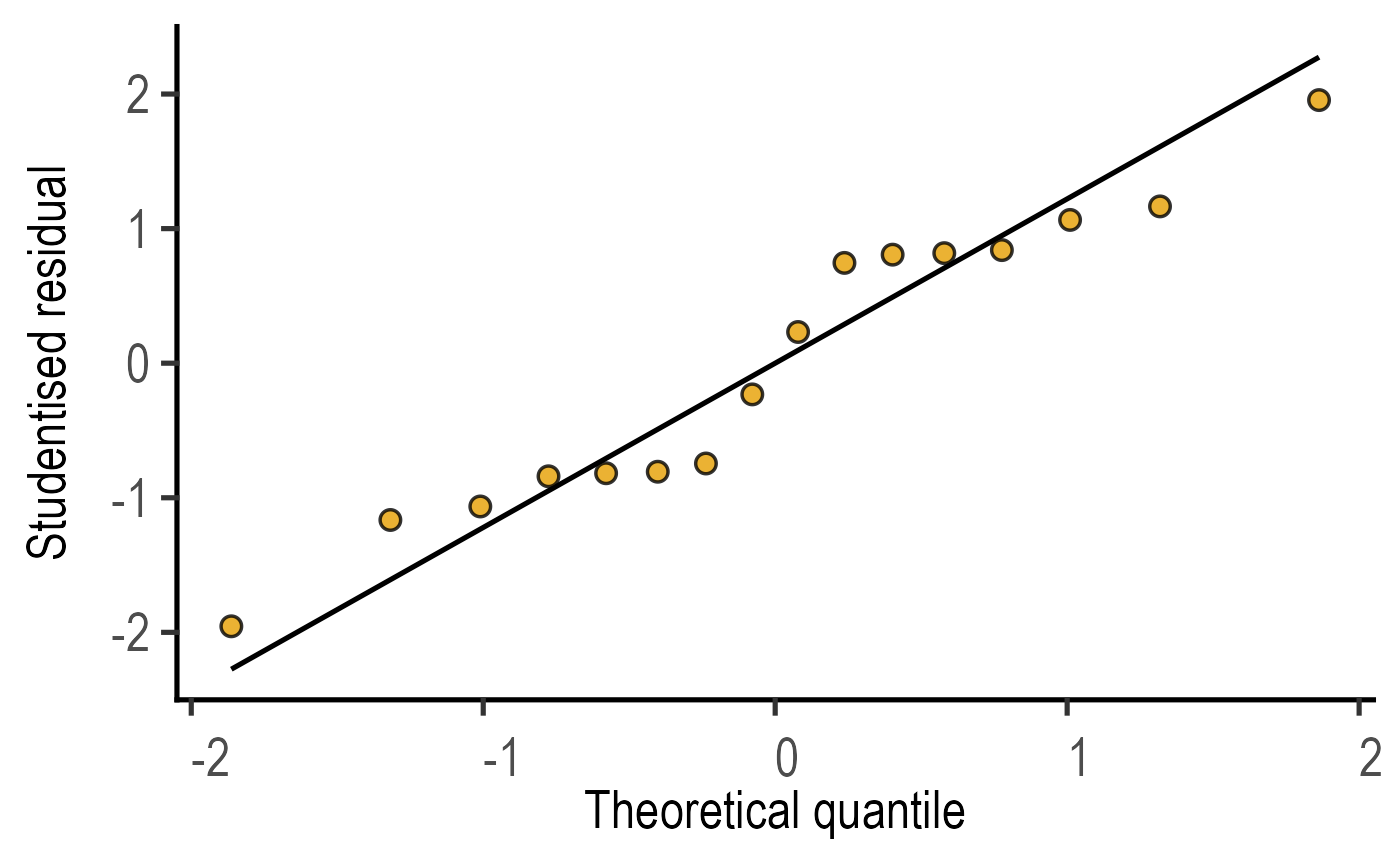This function takes a linear model (simple or mixed effects) and plots a QQ graph after running rstudent from rstudent to generate a table of Studentised model residuals on an ordinary (simple_model), mixed model (mixed_model or mixed_model_slopes. The graph plots studentised residuals from the model (sample) on Y axis & Theoretical quantiles on X axis.

plot_qqmodel(
Model,
symsize = 3,
s_alpha = 0.8,
fontsize = 20,
symthick,
linethick,
SingleColour = "#E69F00"
)

## Arguments

Model

name of a saved model generated by simple_model or mixed_model.

symsize

size of symbols, default set to 3.

s_alpha

fractional opacity of symbols, default set to 0.8 (i.e., 80% opacity).

fontsize

parameter of base_size of fonts in theme_classic, default set to size 20.

symthick

thickness of symbol border, default set to fontsize/22.

linethick

thickness of line, default set to fontsize/22.

SingleColour

colour of symbols (default = #E69F00, which is ok_orange)

## Value

This function returns a ggplot2 object of class "gg" and "ggplot".

## Details

For generalised additive models fit with mgcv, scaled Pearson residuals are plotted.

The function uses ggplot2[geom_qq] and ggplot2[geom_qq_line] geometries. Symbols receive "ok_orange" colour by default.

## Examples

#Basic usage
m1 <- simple_model(data = data_2w_Festing,
Y_value = "GST",
Fixed_Factor = c("Treatment", "Strain"))
plot_qqmodel(m1)